# Lorentz force

In physics (particularly in electromagnetism) the Lorentz force is the combination of electric and magnetic force on a point charge due to electromagnetic fields. If a particle of charge q moves with velocity v in the presence of an electric field E and a magnetic field B, then it will experience a force(in SI units). Variations on this basic formula describe the magnetic force on a current-carrying wire (sometimes called Laplace force), the electromotive force in a wire loop moving through a magnetic field (an aspect of Faraday's law of induction), and the force on a charged particle which might be travelling near the speed of light (relativistic form of the Lorentz force).

The first derivation of the Lorentz force is commonly attributed to Oliver Heaviside in 1889, although other historians suggest an earlier origin in an 1865 paper by James Clerk Maxwell. Hendrik Lorentz derived it a few years after Heaviside.

## Equation (SI units)

### Charged particle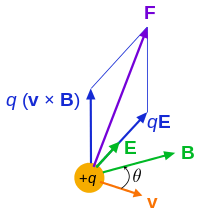Lorentz force F on a charged particle (of charge q) in motion (instantaneous velocity v). The E field and B field vary in space and time.

The force F acting on a particle of electric charge q with instantaneous velocity v, due to an external electric field E and magnetic field B, is given by:where × is the vector cross product. All boldface quantities are vectors. More explicitly stated:in which r is the position vector of the charged particle, t is time, and the overdot is a time derivative.

A positively charged particle will be accelerated in the same linear orientation as the E field, but will curve perpendicularly to both the instantaneous velocity vector v and the B field according to the right-hand rule (in detail, if the fingers of the right hand are extended to point in the direction of v and are then curled to point in the direction of B, then the extended thumb will point in the direction of F).

The term qE is called the electric force, while the term qv × B is called the magnetic force. According to some definitions, the term "Lorentz force" refers specifically to the formula for the magnetic force, with the total electromagnetic force (including the electric force) given some other (nonstandard) name. This article will not follow this nomenclature: In what follows, the term "Lorentz force" will refer only to the expression for the total force.

The magnetic force component of the Lorentz force manifests itself as the force that acts on a current-carrying wire in a magnetic field. In that context, it is also called the Laplace force.

### Continuous charge distribution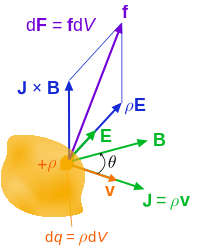Lorentz force (per unit 3-volume) f on a continuous charge distribution (charge density ρ) in motion. The 3-current density J corresponds to the motion of the charge element dq in volume element dV and varies throughout the continuum.

For a continuous charge distribution in motion, the Lorentz force equation becomes:where dF is the force on a small piece of the charge distribution with charge dq. If both sides of this equation are divided by the volume of this small piece of the charge distribution dV, the result is:where f is the force density (force per unit volume) and ρ is the charge density (charge per unit volume). Next, the current density corresponding to the motion of the charge continuum isso the continuous analogue to the equation isThe total force is the volume integral over the charge distribution:By eliminating ρ and J, using Maxwell's equations, and manipulating using the theorems of vector calculus, this form of the equation can be used to derive the Maxwell stress tensor σ, in turn this can be combined with the Poynting vector S to obtain the electromagnetic stress–energy tensor T used in general relativity.

In terms of σ and S, another way to write the Lorentz force (per unit volume) iswhere c is the speed of light and · denotes the divergence of a tensor field. Rather than the amount of charge and its velocity in electric and magnetic fields, this equation relates the energy flux (flow of energy per unit time per unit distance) in the fields to the force exerted on a charge distribution. See Covariant formulation of classical electromagnetism for more details.

## History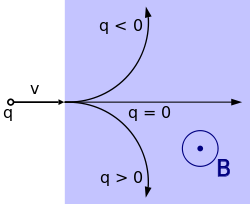Trajectory of a particle with a positive or negative charge q under the influence of a magnetic field B, which is directed perpendicularly out of the screen.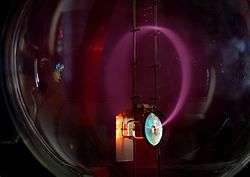Beam of electrons moving in a circle, due to the presence of a magnetic field. Purple light is emitted along the electron path, due to the electrons colliding with gas molecules in the bulb. A Teltron tube is used in this example.
Charged particles experiencing the Lorentz force.

Early attempts to quantitatively describe the electromagnetic force were made in the mid-18th century. It was proposed that the force on magnetic poles, by Johann Tobias Mayer and others in 1760,and electrically charged objects, by Henry Cavendish in 1762, obeyed an inverse-square law. However, in both cases the experimental proof was neither complete nor conclusive. It was not until 1784 when Charles-Augustin de Coulomb, using a torsion balance, was able to definitively show through experiment that this was true. Soon after the discovery in 1820 by H. C. Ørsted that a magnetic needle is acted on by a voltaic current, André-Marie Ampère that same year was able to devise through experimentation the formula for the angular dependence of the force between two current elements. In all these descriptions, the force was always given in terms of the properties of the objects involved and the distances between them rather than in terms of electric and magnetic fields.

The modern concept of electric and magnetic fields first arose in the theories of Michael Faraday, particularly his idea of lines of force, later to be given full mathematical description by Lord Kelvin and James Clerk Maxwell. From a modern perspective it is possible to identify in Maxwell's 1865 formulation of his field equations a form of the Lorentz force equation in relation to electric currents, however, in the time of Maxwell it was not evident how his equations related to the forces on moving charged objects. J. J. Thomson was the first to attempt to derive from Maxwell's field equations the electromagnetic forces on a moving charged object in terms of the object's properties and external fields. Interested in determining the electromagnetic behavior of the charged particles in cathode rays, Thomson published a paper in 1881 wherein he gave the force on the particles due to an external magnetic field asThomson derived the correct basic form of the formula, but, because of some miscalculations and an incomplete description of the displacement current, included an incorrect scale-factor of a half in front of the formula. Oliver Heaviside invented the modern vector notation and applied it to Maxwell's field equations; he also (in 1885 and 1889) had fixed the mistakes of Thomson's derivation and arrived at the correct form of the magnetic force on a moving charged object. Finally, in 1892, Hendrik Lorentz derived the modern form of the formula for the electromagnetic force which includes the contributions to the total force from both the electric and the magnetic fields. Lorentz began by abandoning the Maxwellian descriptions of the ether and conduction. Instead, Lorentz made a distinction between matter and the luminiferous aether and sought to apply the Maxwell equations at a microscopic scale. Using Heaviside's version of the Maxwell equations for a stationary ether and applying Lagrangian mechanics (see below), Lorentz arrived at the correct and complete form of the force law that now bears his name.

## Trajectories of particles due to the Lorentz force

Main article: Guiding center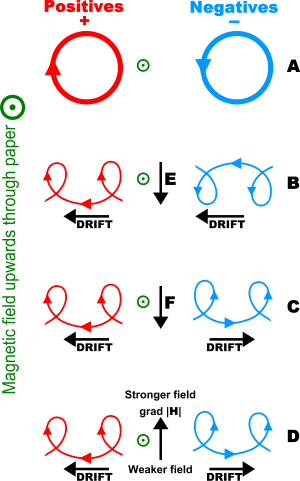Charged particle drifts in a homogeneous magnetic field. (A) No disturbing force (B) With an electric field, E (C) With an independent force, F (e.g. gravity) (D) In an inhomogeneous magnetic field, grad H

In many cases of practical interest, the motion in a magnetic field of an electrically charged particle (such as an electron or ion in a plasma) can be treated as the superposition of a relatively fast circular motion around a point called the guiding center and a relatively slow drift of this point. The drift speeds may differ for various species depending on their charge states, masses, or temperatures, possibly resulting in electric currents or chemical separation.

## Significance of the Lorentz force

While the modern Maxwell's equations describe how electrically charged particles and currents or moving charged particles give rise to electric and magnetic fields, the Lorentz force law completes that picture by describing the force acting on a moving point charge q in the presence of electromagnetic fields. The Lorentz force law describes the effect of E and B upon a point charge, but such electromagnetic forces are not the entire picture. Charged particles are possibly coupled to other forces, notably gravity and nuclear forces. Thus, Maxwell's equations do not stand separate from other physical laws, but are coupled to them via the charge and current densities. The response of a point charge to the Lorentz law is one aspect; the generation of E and B by currents and charges is another.

In real materials the Lorentz force is inadequate to describe the collective behavior of charged particles, both in principle and as a matter of computation. The charged particles in a material medium not only respond to the E and B fields but also generate these fields. Complex transport equations must be solved to determine the time and spatial response of charges, for example, the Boltzmann equation or the Fokker–Planck equation or the Navier–Stokes equations. For example, see magnetohydrodynamics, fluid dynamics, electrohydrodynamics, superconductivity, stellar evolution. An entire physical apparatus for dealing with these matters has developed. See for example, Green–Kubo relations and Green's function (many-body theory).

## Lorentz force law as the definition of E and B

In many textbook treatments of classical electromagnetism, the Lorentz force Law is used as the definition of the electric and magnetic fields E and B. To be specific, the Lorentz force is understood to be the following empirical statement:

The electromagnetic force F on a test charge at a given point and time is a certain function of its charge q and velocity v, which can be parameterized by exactly two vectors E and B, in the functional form:This is valid, even for particles approaching the speed of light (that is, magnitude of v = |v| = c). So the two vector fields E and B are thereby defined throughout space and time, and these are called the "electric field" and "magnetic field". The fields are defined everywhere in space and time with respect to what force a test charge would receive regardless of whether a charge is present to experience the force.

As a definition of E and B, the Lorentz force is only a definition in principle because a real particle (as opposed to the hypothetical "test charge" of infinitesimally-small mass and charge) would generate its own finite E and B fields, which would alter the electromagnetic force that it experiences. In addition, if the charge experiences acceleration, as if forced into a curved trajectory by some external agency, it emits radiation that causes braking of its motion. See for example Bremsstrahlung and synchrotron light. These effects occur through both a direct effect (called the radiation reaction force) and indirectly (by affecting the motion of nearby charges and currents). Moreover, net force must include gravity, electroweak, and any other forces aside from electromagnetic force.

## Force on a current-carrying wire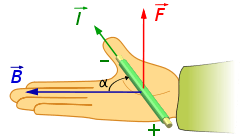Right-hand rule for a current-carrying wire in a magnetic field B

When a wire carrying an electric current is placed in a magnetic field, each of the moving charges, which comprise the current, experiences the Lorentz force, and together they can create a macroscopic force on the wire (sometimes called the Laplace force). By combining the Lorentz force law above with the definition of electric current, the following equation results, in the case of a straight, stationary wire:where is a vector whose magnitude is the length of wire, and whose direction is along the wire, aligned with the direction of conventional current flow I.

If the wire is not straight but curved, the force on it can be computed by applying this formula to each infinitesimal segment of wire d, then adding up all these forces by integration. Formally, the net force on a stationary, rigid wire carrying a steady current I isThis is the net force. In addition, there will usually be torque, plus other effects if the wire is not perfectly rigid.

One application of this is Ampère's force law, which describes how two current-carrying wires can attract or repel each other, since each experiences a Lorentz force from the other's magnetic field. For more information, see the article: Ampère's force law.

## EMF

The magnetic force (q v × B) component of the Lorentz force is responsible for motional electromotive force (or motional EMF), the phenomenon underlying many electrical generators. When a conductor is moved through a magnetic field, the magnetic force tries to push electrons through the wire, and this creates the EMF. The term "motional EMF" is applied to this phenomenon, since the EMF is due to the motion of the wire.

In other electrical generators, the magnets move, while the conductors do not. In this case, the EMF is due to the electric force (qE) term in the Lorentz Force equation. The electric field in question is created by the changing magnetic field, resulting in an induced EMF, as described by the Maxwell–Faraday equation (one of the four modern Maxwell's equations).

Both of these EMF's, despite their different origins, can be described by the same equation, namely, the EMF is the rate of change of magnetic flux through the wire. (This is Faraday's law of induction, see below.) Einstein's special theory of relativity was partially motivated by the desire to better understand this link between the two effects. In fact, the electric and magnetic fields are different faces of the same electromagnetic field, and in moving from one inertial frame to another, the solenoidal vector field portion of the E-field can change in whole or in part to a B-field or vice versa.

## Lorentz force and Faraday's law of induction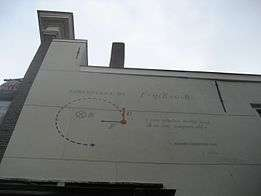Lorentz force -image on a wall in Leiden

Given a loop of wire in a magnetic field, Faraday's law of induction states the induced electromotive force (EMF) in the wire is:whereis the magnetic flux through the loop, B is the magnetic field, Σ(t) is a surface bounded by the closed contour ∂Σ(t), at all at time t, dA is an infinitesimal vector area element of Σ(t) (magnitude is the area of an infinitesimal patch of surface, direction is orthogonal to that surface patch).

The sign of the EMF is determined by Lenz's law. Note that this is valid for not only a stationary wire  but also for a moving wire.

From Faraday's law of induction (that is valid for a moving wire, for instance in a motor) and the Maxwell Equations, the Lorentz Force can be deduced. The reverse is also true, the Lorentz force and the Maxwell Equations can be used to derive the Faraday Law.

Let Σ(t) be the moving wire, moving together without rotation and with constant velocity v and Σ(t) be the internal surface of the wire. The EMF around the closed path ∂Σ(t) is given by:whereis the electric field and d is an infinitesimal vector element of the contour ∂Σ(t).

NB: Both d and dA have a sign ambiguity; to get the correct sign, the right-hand rule is used, as explained in the article Kelvin–Stokes theorem.

The above result can be compared with the version of Faraday's law of induction that appears in the modern Maxwell's equations, called here the Maxwell–Faraday equation:The Maxwell–Faraday equation also can be written in an integral form using the Kelvin–Stokes theorem.

So we have, the Maxwell Faraday equation:The two are equivalent if the wire is not moving. Using the Leibniz integral rule and that div B = 0, results in,and using the Maxwell Faraday equation,since this is valid for any wire position it implies that,Faraday's law of induction holds whether the loop of wire is rigid and stationary, or in motion or in process of deformation, and it holds whether the magnetic field is constant in time or changing. However, there are cases where Faraday's law is either inadequate or difficult to use, and application of the underlying Lorentz force law is necessary. See inapplicability of Faraday's law.

If the magnetic field is fixed in time and the conducting loop moves through the field, the magnetic flux ΦB linking the loop can change in several ways. For example, if the B-field varies with position, and the loop moves to a location with different B-field, ΦB will change. Alternatively, if the loop changes orientation with respect to the B-field, the B • dA differential element will change because of the different angle between B and dA, also changing ΦB. As a third example, if a portion of the circuit is swept through a uniform, time-independent B-field, and another portion of the circuit is held stationary, the flux linking the entire closed circuit can change due to the shift in relative position of the circuit's component parts with time (surface ∂Σ(t) time-dependent). In all three cases, Faraday's law of induction then predicts the EMF generated by the change in ΦB.

Note that the Maxwell Faraday's equation implies that the Electric Field E is non conservative when the Magnetic Field B varies in time, and is not expressible as the gradient of a scalar field, and not subject to the gradient theorem since its rotational is not zero.

## Lorentz force in terms of potentials

The E and B fields can be replaced by the magnetic vector potential A and (scalar) electrostatic potential ϕ bywhere ∇ is the gradient, ∇• is the divergence, ∇ × is the curl.

The force becomesand using an identity for the triple product simplifies tousing the chain rule, the total derivative of A is:so the above expression can be rewritten as;which can take the convenient Euler–Lagrange form## Lorentz force and analytical mechanics

The Lagrangian for a charged particle of mass m and charge q in an electromagnetic field equivalently describes the dynamics of the particle in terms of its energy, rather than the force exerted on it. The classical expression is given by:where A and ϕ are the potential fields as above. Using Lagrange's equations, the equation for the Lorentz force can be obtained.

The potential energy depends on the velocity of the particle, so the force is velocity dependent, so it is not conservative.

The relativistic Lagrangian isThe action is the relativistic arclength of the path of the particle in space time, minus the potential energy contribution, plus an extra contribution which quantum mechanically is an extra phase a charged particle gets when it is moving along a vector potential.

## Equation (cgs units)

The above-mentioned formulae use SI units which are the most common among experimentalists, technicians, and engineers. In cgs-Gaussian units, which are somewhat more common among theoretical physicists, one has insteadwhere c is the speed of light. Although this equation looks slightly different, it is completely equivalent, since one has the following relations:where ε0 is the vacuum permittivity and μ0 the vacuum permeability. In practice, the subscripts "cgs" and "SI" are always omitted, and the unit system has to be assessed from context.

## Relativistic form of the Lorentz force

### Covariant form of the Lorentz force

#### Field tensor

Using the metric signature (1, −1, −1, −1), The Lorentz force for a charge q can be written in covariant form:where pα is the four-momentum, defined asτ the proper time of the particle, Fαβ the contravariant electromagnetic tensorand U is the covariant 4-velocity of the particle, defined as:in whichis the Lorentz factor.

The fields are transformed to a frame moving with constant relative velocity by:where Λμα is the Lorentz transformation tensor.

#### Translation to vector notation

The α = 1 component (x-component) of the force isSubstituting the components of the covariant electromagnetic tensor F yieldsUsing the components of covariant four-velocity yieldsThe calculation for α = 2, 3 (force components in the y and z directions) yields similar results, so collecting the 3 equations into one:and since differentials in coordinate time dt and proper time are related by the Lorentz factor,so we arrive atThis is precisely the Lorentz force law, however, it is important to note that p is the relativistic expression,### Lorentz force in spacetime algebra (STA)

The electric and magnetic fields are dependent on the velocity of an observer, so the relativistic form of the Lorentz force law can best be exhibited starting from a coordinate-independent expression for the electromagnetic and magnetic fields, and an arbitrary time-direction,. This can be settled through Space-Time Algebra (or the geometric algebra of space-time), a type of Clifford's Algebra defined on a pseudo-euclidian space, asandis a space-time bivector (an oriented plane segment, just like a vector is an oriented line segment), which has six degrees of freedom corresponding to boosts (rotations in space-time planes) and rotations (rotations in space-space planes). The dot product with the vectorpulls a vector (in the space algebra) from the translational part, while the wedge-product creates a trivector (in the space algebra) who is dual to a vector which is the usual magnetic field vector. The relativistic velocity is given by the (time-like) changes in a time-position vector, where(which shows our choice for the metric) and the velocity isThe proper (invariant is an inadequate term because no transformation has been defined) form of the Lorentz force law is simplyNote that the order is important because between a bivector and a vector the dot product is anti-symmetric. Upon a space time split like one can obtain the velocity, and fields as above yielding the usual expression.

## Applications

The Lorentz force occurs in many devices, including:

In its manifestation as the Laplace force on an electric current in a conductor, this force occurs in many devices including:

## Footnotes

1. Huray, Paul G. (2009). Maxwell's Equations. Wiley-IEEE. p. 22. ISBN 0-470-54276-4.
2. See Jackson page 2. The book lists the four modern Maxwell's equations, and then states, "Also essential for consideration of charged particle motion is the Lorentz force equation, F = q ( E+ v × B ), which gives the force acting on a point charge q in the presence of electromagnetic fields."
3. See Griffiths page 204.
4. For example, see the website of the "Lorentz Institute": \, or Griffiths.
5. Griffiths, David J. (1999). Introduction to electrodynamics. reprint. with corr. (3rd ed.). Upper Saddle River, New Jersey [u.a.]: Prentice Hall. ISBN 978-0-13-805326-0.
6. Delon, Michel (2001). Encyclopedia of the Enlightenment. Chicago, IL: Fitzroy Dearborn Publishers. p. 538. ISBN 157958246X.
7. Goodwin, Elliot H. (1965). The New Cambridge Modern History Volume 8: The American and French Revolutions, 1763–93. Cambridge: Cambridge University Press. p. 130. ISBN 9780521045469.
8. Meyer, Herbert W. (1972). A History of Electricity and Magnetism. Norwalk, Connecticut: Burndy Library. pp. 30–31. ISBN 0-262-13070-X.
9. Verschuur, Gerrit L. (1993). Hidden Attraction : The History And Mystery Of Magnetism. New York: Oxford University Press. pp. 78–79. ISBN 0-19-506488-7.
10. Darrigol, Olivier (2000). Electrodynamics from Ampère to Einstein. Oxford, [England]: Oxford University Press. pp. 9, 25. ISBN 0-19-850593-0.
11. Verschuur, Gerrit L. (1993). Hidden Attraction : The History And Mystery Of Magnetism. New York: Oxford University Press. p. 76. ISBN 0-19-506488-7.
12. Darrigol, Olivier (2000). Electrodynamics from Ampère to Einstein. Oxford, [England]: Oxford University Press. pp. 126–131, 139–144. ISBN 0-19-850593-0.
13. Darrigol, Olivier (2000). Electrodynamics from Ampère to Einstein. Oxford, [England]: Oxford University Press. pp. 200, 429–430. ISBN 0-19-850593-0.
14. Heaviside, Oliver. "On the Electromagnetic Effects due to the Motion of Electrification through a Dielectric". Philosophical Magazine, April 1889, p. 324.
15. Darrigol, Olivier (2000). Electrodynamics from Ampère to Einstein. Oxford, [England]: Oxford University Press. p. 327. ISBN 0-19-850593-0.
16. Whittaker, E. T. (1910). A History of the Theories of Aether and Electricity: From the Age of Descartes to the Close of the Nineteenth Century. Longmans, Green and Co. pp. 420–423. ISBN 1-143-01208-9.
17. See Griffiths page 326, which states that Maxwell's equations, "together with the [Lorentz] force law...summarize the entire theoretical content of classical electrodynamics".
18. See, for example, Jackson pp 777–8.
19. J.A. Wheeler; C. Misner; K.S. Thorne (1973). Gravitation. W.H. Freeman & Co. pp. 72–73. ISBN 0-7167-0344-0.. These authors use the Lorentz force in tensor form as definer of the electromagnetic tensor F, in turn the fields E and B.
20. I.S. Grant; W.R. Phillips; Manchester Physics (2008). Electromagnetism (2nd ed.). John Wiley & Sons. p. 122. ISBN 978-0-471-92712-9.
21. I.S. Grant; W.R. Phillips; Manchester Physics (2008). Electromagnetism (2nd Edition). John Wiley & Sons. p. 123. ISBN 978-0-471-92712-9.
22. See Griffiths pages 301–3.
23. Tai L. Chow (2006). Electromagnetic theory. Sudbury MA: Jones and Bartlett. p. 395. ISBN 0-7637-3827-1.
24. Landau, L. D., Lifshit︠s︡, E. M., & Pitaevskiĭ, L. P. (1984). Electrodynamics of continuous media; Volume 8 Course of Theoretical Physics (Second ed.). Oxford: Butterworth-Heinemann. p. §63 (§49 pp. 205–207 in 1960 edition). ISBN 0-7506-2634-8.
25. Roger F Harrington (2003). Introduction to electromagnetic engineering. Mineola, New York: Dover Publications. p. 56. ISBN 0-486-43241-6.
26. M N O Sadiku (2007). Elements of electromagnetics (Fourth ed.). NY/Oxford: Oxford University Press. p. 391. ISBN 0-19-530048-3.
27. Classical Mechanics (2nd Edition), T.W.B. Kibble, European Physics Series, Mc Graw Hill (UK), 1973, ISBN 0-07-084018-0.
28. Jackson, J.D. Chapter 11

The numbered references refer in part to the list immediately below.

• Feynman, Richard Phillips; Leighton, Robert B.; Sands, Matthew L. (2006). The Feynman lectures on physics (3 vol.). Pearson / Addison-Wesley. ISBN 0-8053-9047-2.: volume 2.
• Griffiths, David J. (1999). Introduction to electrodynamics (3rd ed.). Upper Saddle River, [NJ.]: Prentice-Hall. ISBN 0-13-805326-X.
• Jackson, John David (1999). Classical electrodynamics (3rd ed.). New York, [NY.]: Wiley. ISBN 0-471-30932-X.
• Serway, Raymond A.; Jewett, John W., Jr. (2004). Physics for scientists and engineers, with modern physics. Belmont, [CA.]: Thomson Brooks/Cole. ISBN 0-534-40846-X.
• Srednicki, Mark A. (2007). Quantum field theory. Cambridge, [England] ; New York [NY.]: Cambridge University Press. ISBN 978-0-521-86449-7.Wikimedia Commons has media related to Lorentz force.